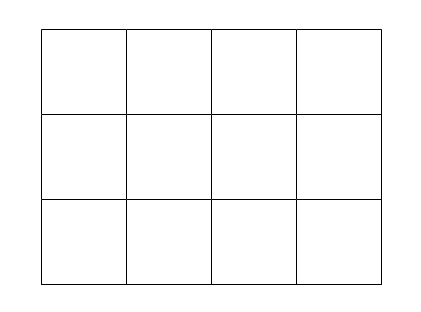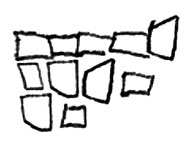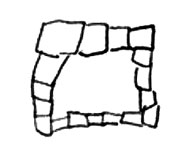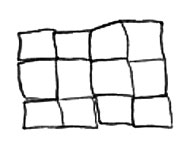Home > Patterns > Misunderstandings > Rectangular grids > Structure of rectangular grids

# Structure of rectangular grids

Some year 1 students were given square tiles and asked to make a 3 $$\times$$ 4 rectangular grid, like the one below, and then draw it.A 3 $$\times$$ 4 rectangular grid.

They easily made the grid, but had difficulty drawing it. Here are some of their drawings.Ashah's drawing.Bonney's drawing.Con's drawing.

Ashah knew that the grid was made of squares and had some idea that they were arranged in rows, but she could not complete the pattern.

Bonny concentrated on the squares around the edge of the grid, but did not have the correct number of squares.

Only Con drew the structure correctly, but she drew each square separately instead of using straight lines to show the rows and columns.

These drawings are typical of young students. They suggest that many do not fully understand the row-column structure of the rectangular array. They may therefore have some difficulty understanding its use to illustrate multiplication, area or fractions.